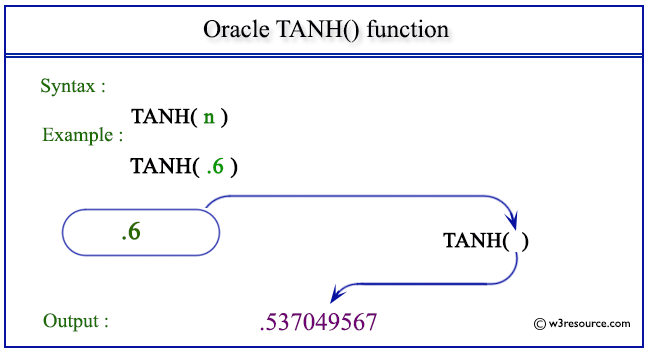# Oracle TANH() function

## Description

This TANH() function returns the hyperbolic tangent of a given number.
The function takes any numeric or nonnumeric data type (can be implicitly converted to a numeric data type) as an argument.
If the argument is BINARY_FLOAT, then the function returns BINARY_DOUBLE. Otherwise, the function returns the same numeric data type as the argument

Syntax:

`TAN(X)`

Where X is a number

Pictorial Presentation of TANH() functionExample - 1

``````SELECT TANH(.6) "Hyperbolic tangent of .6" FROM dual;
```
```

Here is the result.

```Hyperbolic tangent of .6
------------------------
.537049567
```

The above statement will return the tangent of the given number .6

Previous: TAN
Next: TRUNC

﻿